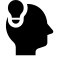# 贝叶斯网络

## Bayesian Network

Posted by viewsetting on May 22, 2019

# 动机

Bayesian Network的定义是：

1. 每个节点就是一个随机变量，可以是连续也可以为离散
2. DAG，即有向无环图。如果$X\rightarrow Y$ ,则称$X$为$Y$的父节点
3. 每个节点$X_i$都有一个条件概率分布$P(X_i\vert Parents(X_i))$

## 分解因式

$P(x_1,x_2,…,x_n)=\prod_{k=1}^nP(x_k\vert x_{1:k-1})$

$P(x_1,x_2,…,x_n)=\prod_{i=1}^pP(x_i\vert x_{parent(i)})$

# 三种分解后的模式

Union Distribution:

$P(a,b,c)=P(a)P(b\vert a)P(c\vert a,b)$

Bayesian Rule:

$P(a,b,c)=P(a)P(b\vert a)P(c\vert a)$

$P(c\vert a,b)=P(c\vert a)$,故在给定$P(a)$时，$b \perp c \vert a$ 即$b$和$c$在给定$a$的概率时（观测到$a$的概率）条件独立。

### tail2tail

$P(a,b,c)=P(a)P(b\vert a)P(c\vert a,b)$

$P(a,b,c)=P(a)P(b)P(c\vert a,b)$

# 应用

### D-Separation

If we have 3 sets $X_A,X_B,X_C$, all of which are consists of randomized varieties and the intersections between each are empty sets.

Assume that there is a path between nodes of $X_A$to those of $X_C$,all of nodes on that path should be in $X_B$.

If there is $X_B\rightarrow X_A$ and $X_B\rightarrow X_C$ ，with $X_B$ be observed, we will have the conclusion $X_A\perp X_C \vert X_B$

### Markov Blanket

• $x_i$的父节点
• $x_i$的子节点
• $x_i$所有子节点的所有除了$x_i$的父节点

$=P(X)/ \int_{x_i} P(x) dx_i$

# 贝叶斯网络分类

### 单一模型

Naive Bayes, 基于朴素贝叶斯假设：$P(x\vert y)=\prod_{i=1}^nP(x_i\vert y=1)$

### 混合模型

Gaussian Mixed Model，可用于Clustering。

### 基于时间的模型

Markov Chain

HMM , 隐马尔科夫模型。

Gaussian Process 高斯过程，无限维的Gaussian Distribution

LDS 线性动态系统 如：Kalman Filter

Particle Filter 非连续非线性系统

### 连续模型

Gaussian Bayesian Network